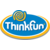# Math Dice Jr. Game#### Code: 45151527

C\$13.99

Out of stock

Math Dice® Jr.
A Dice Game that Makes Math Fun!
Math Dice Jr. is the perfect dice game to introduce or reinforce early math skills in children. Roll the 12-sided Target Die to get your target number, then roll the five 6-sided Scoring Dice. Add, subtrac...Read more

# Math Dice® Jr.

A Dice Game that Makes Math Fun!
Math Dice Jr. is the perfect dice game to introduce or reinforce early math skills in children. Roll the 12-sided Target Die to get your target number, then roll the five 6-sided Scoring Dice. Add, subtract, or add and subtract – use whatever equation you need to hit your target number. For every Scoring Die used, move one space on the Scoring Track. First to the finish line wins! Math Dice Jr. makes math fun.

Ages 6+

For 2 or more players